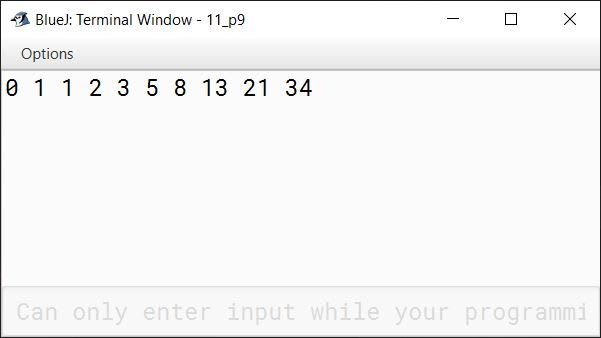### icse board class 8 programs

This group contains icse board class 8 programs .

## Write a program to print the Fibonacci series upto 10 terms.

Write a program to print the Fibonacci series upto 10 terms.
[A series of numbers in which each number is the sum of the two preceding numbers. For example: 0,1,1,2,3, ............... n].

```public class KboatFibonacci
{
public static void main(String args[]) {
int a = 0;
int b = 1;
System.out.print(a + " " + b);
/*
* i is starting from 3 below
* instead of 1 because we have
* already printed 2 terms of
* the series. The for loop will
* print the series from third
* term onwards.
*/
for (int i = 3; i <= 10; i++) {
int term = a + b;
System.out.print(" " + term);
a = b;
b = term;
}
}
}```
##### Output